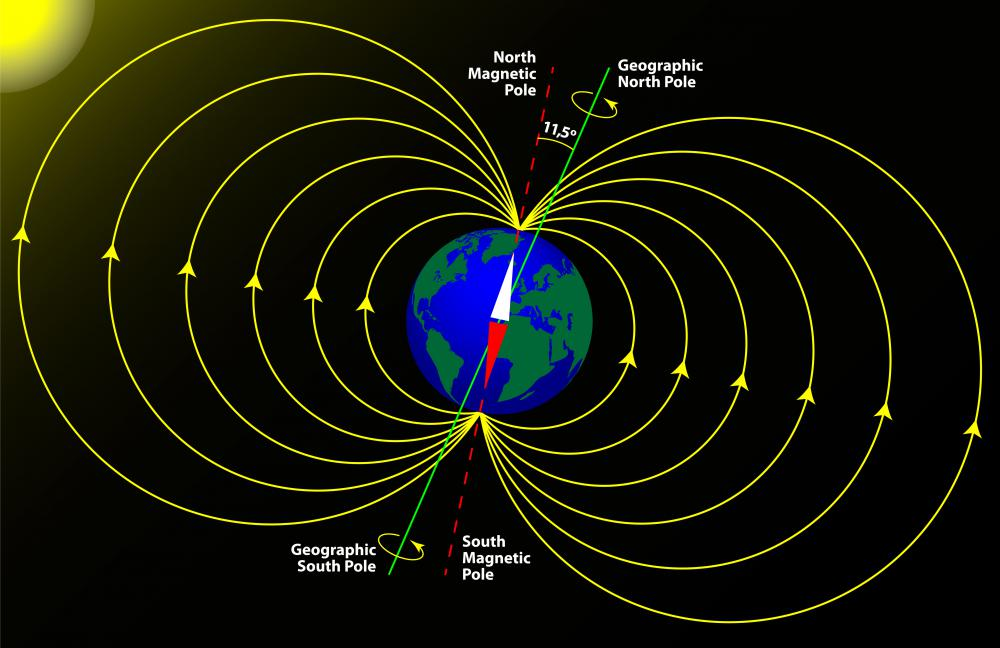# What Is a Magnetic Dipole?

E. Starr
E. Starr

A magnetic dipole can be considered the fundamental observable unit of magnetism. Most intuitively, a dipole consists of two equal but oppositely charged points, or poles. The interaction between these two charged monopoles generates a vector field of force in the surrounding area known as a magnetic field. Familiar examples of magnetic dipoles include bar magnets, electrons and the planet Earth itself.The Earth's magnetic field is an example of a dipole field.

Although it is often simplest to consider magnetic materials such as bar magnets as having two monopoles of electric charge, this model is unsuccessful at describing the behavior within a magnet. Additionally, monopoles have never actually been observed. Rather, monopoles are hypothetical particles. Interestingly, the existence of monopoles has been postulated by theoretical physics, and the existence and nature of monopoles has been an active open question in science.

A second model with which one might consider magnetic dipoles is that of current loops. Hans Christian Oersted discovered in 1820 that a closed electric circuit, or closed loop of current, generates a magnetic field. He did this by placing an electrically charged wire near a compass and noticing that the compass needle moved. The current loop was creating a magnetic field that influenced the magnetic needle, or dipole, within the compass. The dipole of a magnetic material such as a bar magnet can be modeled by envisioning the structure as being filled with small current loops. Models using these current loops do predict the behavior within magnetic materials with great success.

The strength of a dipole is measured as the magnetic dipole moment. The moment is a vector, meaning it has a magnitude, or size, as well as a direction. When considering magnetic poles, such as those in a bar magnet, the magnetic moment (m) is defined as the strength of the poles (p) multiplied by the distance between the poles (L), which can be represented by the equation m = pL. The direction of the moment points from the south pole of the magnet to its north pole.

The magnetic moment also can be defined for a magnetic dipole created by an electric current. In this case, the magnetic moment is equal to the current (I) multiplied by the area within the current loop (s), which can be represented by the equation m = Is. The direction of this moment can be determined by the right-hand rule. To use this rule, a person holds his or her right hand to the front and lets his or her fingers curve or close into a fist in the same direction as the current. The person's right thumb, if held straight, will point in the direction of the magnetic dipole moment.

## You might also Like